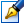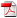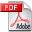##A semi-isometric isomorphism of pseudonormed rings and its properties

 Author: Alioscenco Svetlana Degree: doctor of physics and mathematics Speciality: 01.01.06 - Mathematical logics, algebra and theory of numbers Year: 2016 Scientific adviser: Vladimir Arnautovdoctor habilitat, professor, Institute of Mathematics and Computer Science Institution: Tiraspol State University

### Status

The thesis was presented on the 17 May, 2016
Approved by NCAA on the 5 July, 2016

### Abstract0.27 Mb / in romanian

### Thesis

CZU 512.556.31.45 Mb / in russian
137 pages

### Keywords

pseudonormed ring, ideal, quotient ring, subring, one-sided ideal, accessible subring, isometric homomorphism, semi-isometric isomorphism

### Summary

Thesis structure: the thesis is written in Russian and consists of an introduction, three chapters, general conclusions and recommendations, bibliography of 85 titles, 127 pages of main text. The obtained results are published in 15 scientific papers.
Field of study of the thesis: belongs to study of properties of homomorphisms and isomorphisms of pseudonormed rings and their applications.
Thesis aim and objectives: – determination of classes of special isomorphisms of pseudonormed rings for which analogues of the second isomorphism theorem hold;
– study of properties of special isomorphisms of pseudonormed rings;
– study of constructions of pseudonormed rings which are preserving special isomorphisms.

Scientific novelty and originality:
– were definited concepts of a semi-isometric isomorphism (semi-isometric isomorphism on the left, semi-isometric isomorphism on the right) of pseudonormed rings;
– were proved criterions of a semi-isometric isomorphism (semi-isometric isomorphism on the left, semi-isometric isomorphism on the right);
– were established some properties of a semi-isometric isomorphism (semi-isometric isomorphism on the left, semi-isometric isomorphism on the right);
– were proved theorems about preservation of a semi-isometric isomorphism (semiisometric isomorphism on the left, semi-isometric isomorphism on the right) for some constructions of pseudonormed rings.

The important scientific solved problem consists of determination of classes of special isomorphisms of pseudonormed rings for which analogues of the second isomorphism theorem hold and study of their properties which led to study of constructions of pseudonormed rings which are preserving these isomorphisms.

The theoretical significance and applicative value of the thesis consists in development of research methods for isomorphisms of pseudonormed rings on semi-isometry (semi-isometry on the left, semi-isometry on the right), establishment of sufficient conditions of preservation of semi-isometric isomorphism (semi-isometric isomorphism on the left, semi-isometric isomorphism on the right) for some constructions of pseudonormed rings.

The implementation of the scientific results:
– the results and methods which are developed in the thesis can be applied for further investigations of isomorphisms and homomorphisms of pseudonormed rings and Banach rings;
– the results of the thesis can be applied to the construction of the general theory of radicals of pseudonormed rings;
– the results of the thesis can serve as support for master thesis and can be used as content for some special courses of undergraduate and graduate programs for students of mathematical specialties.